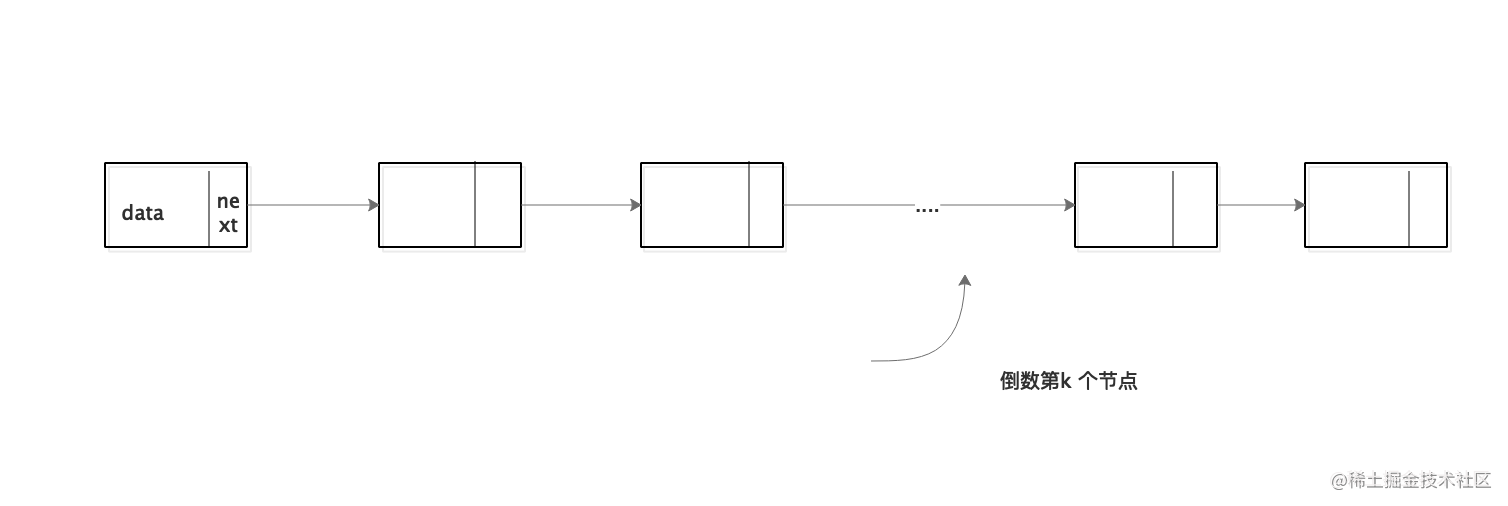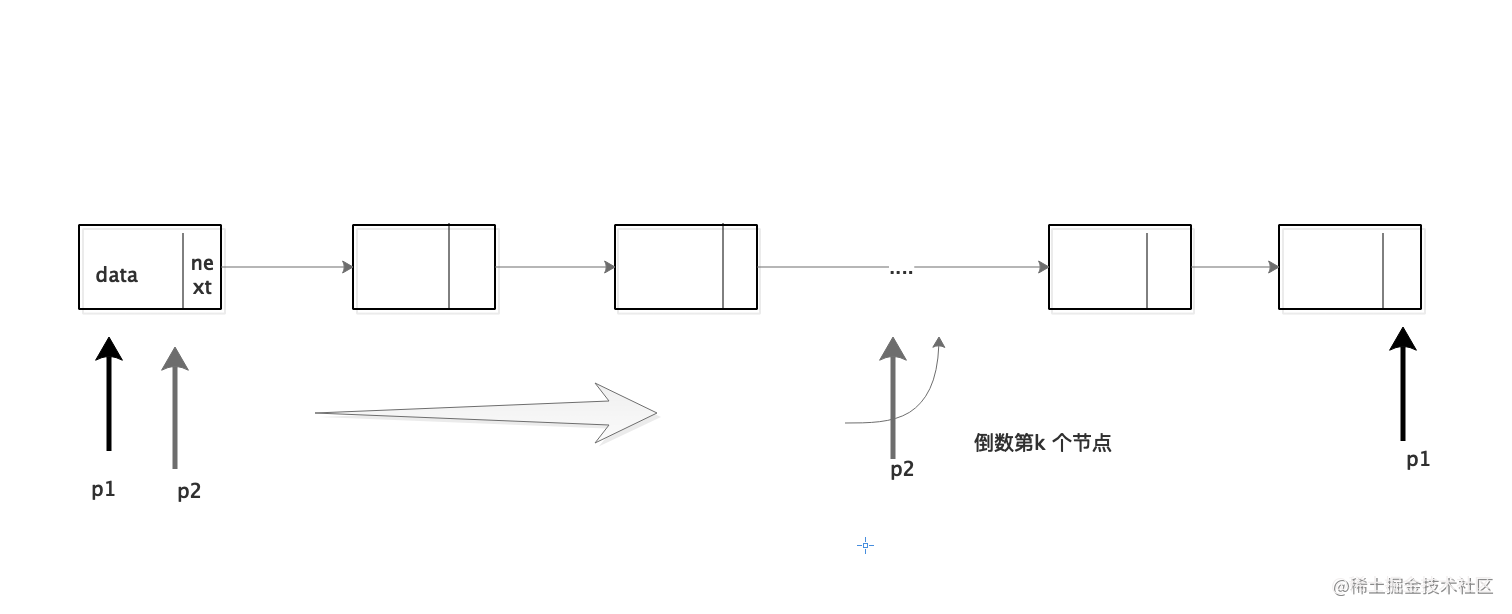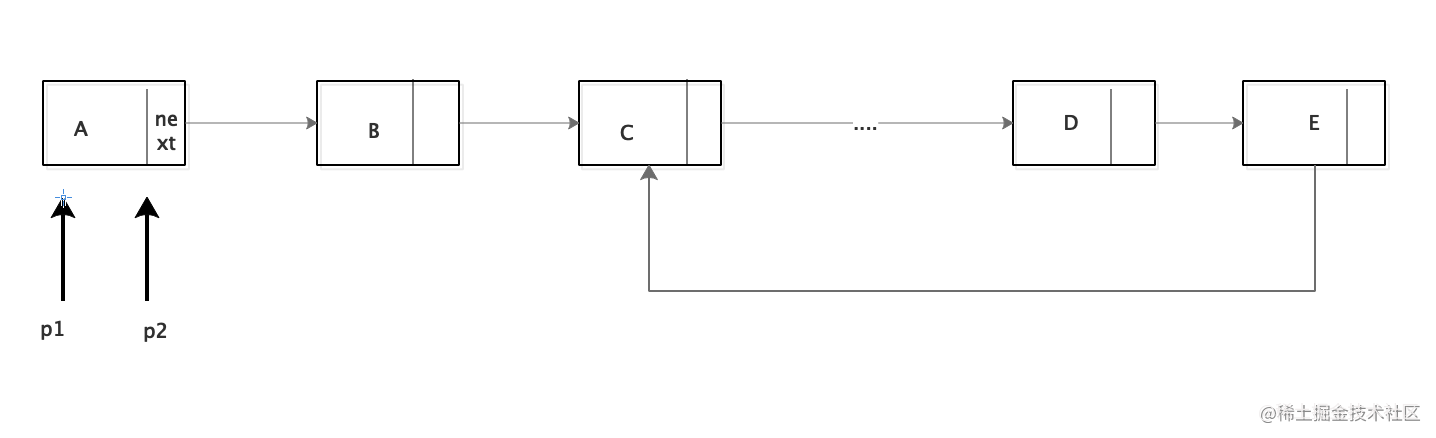# 連結串列演算法經典題

theme: channing-cyan highlight: a11y-light

```/*連結串列*/struct ListNode { int data; struct ListNode *next; struct ListNode *pre; };```

```struct ListNode* constructList(void) { //頭結點定義 struct ListNode *head = NULL; //記錄當前結點 struct ListNode *cur = NULL; for (int i = 0; i < 8; i++) { struct ListNode *node = malloc(sizeof(struct ListNode)); node->data = i; //頭結點為空,新結點即為頭結點 if (head == NULL) { head = node; } else {//當前結點的next為新結點 cur ->next = node; } //設定當前結點為新結點 cur = node; } return head; }```

``` struct ListNode constructCycleList(void) { //頭結點定義 struct ListNode head = NULL; //記錄當前尾結點 struct ListNode cur = NULL; //環結點入口 struct ListNode cycle = NULL;

``````for (int i = 0; i < 8; i++) {
struct ListNode *node = malloc(sizeof(struct ListNode));
node->data = i;
if (i == 3) {//第4個為環入口
cycle = node;
}
//頭結點為空,新結點即為頭結點
} else {//當前結點的next為新結點
cur->next = node;
}
//設定當前結點為新結點
cur = node;
if (i == 7) {
cur->next = cycle;
}
}
``````

} ```

### 1. 連結串列的倒數第K個節點```struct ListNode* findKth(struct ListNode *head, int k) { struct ListNode* cur = head; struct ListNode* now = head; int i = 0; while (cur!=NULL&&i++<k) { cur = cur->next; } while (cur!=NULL) { now =now->next; cur =cur->next; } return now; }```

### 2.從尾到頭列印連結串列（遞迴和非遞迴）

```struct ListNode* reverseList(struct ListNode* head) { if (head == NULL || head->next == NULL) return head; struct ListNode *nextNode = head->next; struct ListNode *reverseNode =reverseList(nextNode); nextNode->next = head; head->next = NULL; return reverseNode; }```

### 3.如何判斷一個連結串列有環### 4.連結串列中環的大小

``` //首先找出相遇時候的環節點,然後再次到此節點則表示環大小 struct ListNode cycleNode(struct ListNode head) { //使用快慢指標，慢指標每次向前一步，快指標每次兩步 struct ListNode slowPoint = head; struct ListNode fastPoint = head; while (fastPoint != NULL && fastPoint->next != NULL) { slowPoint = slowPoint->next; fastPoint = fastPoint->next; if (fastPoint->next != NULL) { fastPoint = fastPoint->next; //兩指標相遇則有環 if (slowPoint == fastPoint) { return slowPoint; } } } return NULL; }

``````struct ListNode *node = cycleNode(head);
//node為空則代表連結串列無環
if (node == NULL) {
return 0;
}
int length = 1;
struct ListNode* currentNode = node->next;
//再次相遇則迴圈結束
while (currentNode != node) {
length++;
currentNode = currentNode->next;
}
printf("cycle length is %d",length);
return length;
``````

} ```

### 5.連結串列中環的入口結點

```struct ListNode* cycleListFirstNode(struct ListNode* head) { struct ListNode* cur = head; struct ListNode* now = head; int cycleLength = getCycleLength(head); int i = 0; while (cur != NULL && i++ < cycleLength) { cur = cur->next; } while (cur != now) { now = now->next; cur = cur->next; } printf("cycle 入口 node value is %d",now->data); return now; }```

「其他文章」